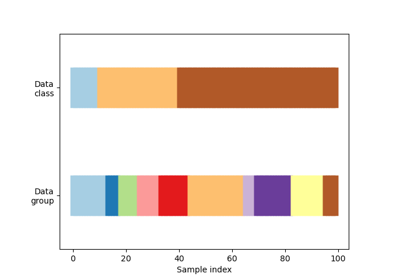# sklearn.model_selection.GroupShuffleSplit¶

class sklearn.model_selection.GroupShuffleSplit(n_splits=5, *, test_size=None, train_size=None, random_state=None)[source]

Shuffle-Group(s)-Out cross-validation iterator

Provides randomized train/test indices to split data according to a third-party provided group. This group information can be used to encode arbitrary domain specific stratifications of the samples as integers.

For instance the groups could be the year of collection of the samples and thus allow for cross-validation against time-based splits.

The difference between LeavePGroupsOut and GroupShuffleSplit is that the former generates splits using all subsets of size p unique groups, whereas GroupShuffleSplit generates a user-determined number of random test splits, each with a user-determined fraction of unique groups.

For example, a less computationally intensive alternative to LeavePGroupsOut(p=10) would be GroupShuffleSplit(test_size=10, n_splits=100).

Note: The parameters test_size and train_size refer to groups, and not to samples, as in ShuffleSplit.

Read more in the User Guide.

Parameters
n_splitsint, default=5

Number of re-shuffling & splitting iterations.

test_sizefloat, int, default=0.2

If float, should be between 0.0 and 1.0 and represent the proportion of groups to include in the test split (rounded up). If int, represents the absolute number of test groups. If None, the value is set to the complement of the train size. The default will change in version 0.21. It will remain 0.2 only if train_size is unspecified, otherwise it will complement the specified train_size.

train_sizefloat or int, default=None

If float, should be between 0.0 and 1.0 and represent the proportion of the groups to include in the train split. If int, represents the absolute number of train groups. If None, the value is automatically set to the complement of the test size.

random_stateint, RandomState instance or None, default=None

Controls the randomness of the training and testing indices produced. Pass an int for reproducible output across multiple function calls. See Glossary.

Examples

>>> import numpy as np
>>> from sklearn.model_selection import GroupShuffleSplit
>>> X = np.ones(shape=(8, 2))
>>> y = np.ones(shape=(8, 1))
>>> groups = np.array([1, 1, 2, 2, 2, 3, 3, 3])
>>> print(groups.shape)
(8,)
>>> gss = GroupShuffleSplit(n_splits=2, train_size=.7, random_state=42)
>>> gss.get_n_splits()
2
>>> for train_idx, test_idx in gss.split(X, y, groups):
...     print("TRAIN:", train_idx, "TEST:", test_idx)
TRAIN: [2 3 4 5 6 7] TEST: [0 1]
TRAIN: [0 1 5 6 7] TEST: [2 3 4]


Methods

 get_n_splits([X, y, groups]) Returns the number of splitting iterations in the cross-validator split(X[, y, groups]) Generate indices to split data into training and test set.
get_n_splits(X=None, y=None, groups=None)[source]

Returns the number of splitting iterations in the cross-validator

Parameters
Xobject

Always ignored, exists for compatibility.

yobject

Always ignored, exists for compatibility.

groupsobject

Always ignored, exists for compatibility.

Returns
n_splitsint

Returns the number of splitting iterations in the cross-validator.

split(X, y=None, groups=None)[source]

Generate indices to split data into training and test set.

Parameters
Xarray-like of shape (n_samples, n_features)

Training data, where n_samples is the number of samples and n_features is the number of features.

yarray-like of shape (n_samples,), default=None

The target variable for supervised learning problems.

groupsarray-like of shape (n_samples,)

Group labels for the samples used while splitting the dataset into train/test set.

Yields
trainndarray

The training set indices for that split.

testndarray

The testing set indices for that split.

Notes

Randomized CV splitters may return different results for each call of split. You can make the results identical by setting random_state to an integer.

## Examples using sklearn.model_selection.GroupShuffleSplit¶